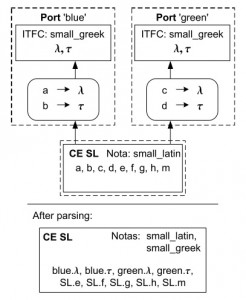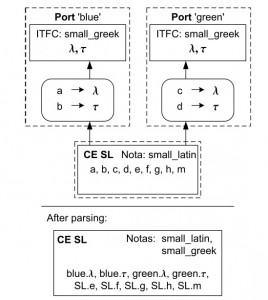# Ports and Streams I – Defining units by adding ports

Stefan Kuntsche, Sanam Sabeti Adibpour, Gregor Tolksdorf

In this tutorial section it is assumed that the parts Connection techniques I – naming policy ‘integrate’ and Connection techniques II – naming policy ‘encapsulate’ have been worked through.

In this section the use of ports in MOSAICmodeling is introduced. Ports are useful to define standardized input and output variables and make equation systems more usable like a unit in the process systems engineering sense.

In MOSAICmodeling there is no fixed specification of the variables in a port or stream. In fact ports and streams can be defined by the modeler and the variable definitions used therein are reusable.

The small example given above is not related to process systems engineering and consequently it does not use any physical values. To make the concepts more transparent, however, some variables are related to colors.

#### Preparations

Example equation system (colors) The following equation system and notation are used as a starting point for this tutorial. As you will see colors are used in the description of the variables.

Equation system ‘eqsys small latin’

• Notation: ‘nota small latin’
• Equations:

Equation ‘eq small latin one’:
$latex a+e\cdot b + f\cdot (c)^{2} = \frac{g}{d}$

Equation ‘eq small latin two’:
$latex h\cdot a + m \cdot (b)^{3} = d$

Notation ‘nota small latin’

• Base Names

[table sort=”desc,asc”]
Name,Description
a,light blue value
b,dark blue value
c,light green value
d,dark green value
e,yellow value
f,pink value
g,light gray value
h,dark gray value
m,black value
[/table]

• Create notation ‘nota small latin’ and ‘eqsys small latin’. When creating the notation, please use the colors given in the description as they will help to intuitively distinguish the variables later.

#### Standardization via modular Interfaces

As mentioned above, the specification of the variables contained in a port or stream must be defined by the user. This is done by the help of a model element called Interface. An Interface contains a list of variables and a Notation defining their meaning. Such an interface can is used in ports as a modular means of standardization.

Motivating example An Interface for a chemical engineering unit could contain the variables F, z_{i}, P, T and w. The notation for such an interface would explain F as molar feed stream, z_{i} as molar fraction of the composition (phase independent), P as pressure, T as temperature, and w as vapor fraction. This interface can be used in ports for units that define material streams with the above mentioned contents.

Continuing the tutorial example (colors) In the tutorial example we want define an interface for light and dark color values. We will use the interface for the equation system ‘eqsys small latin’ created above, to demonstrate the generality of the concept we use a different notation in the interface.

Interface ‘itfc small greek’

• Notation: ‘nota small greek’
• Variables

\lambda, \tau

Notation ‘nota small greek’

• Base Names

[table sort=”desc,asc”]
Name,Description
\lambda,light color value
\tau,dark color value
[/table]

Work flow to create the interface

• Create notation 'nota small greek'.
• Select the Interface Editor tab from the Editor bar.
• Load notation 'nota small greek' in the Notation file panel.
• Below the table Variable Naming press [Add] to open the 'Create Variable Naming' dialog.
• Create the Variable Naming ‘lambda’ (latex: \lambda) by typing ‘\lambda’ followed by clicks on 'Render' [Shift+Enter] and 'OK' [Ctrl+Enter].
• Create ‘tau’ (latex: \tau) in the same way ([Alt+a],’\tau’,[Shift+Enter],[Ctrl+Enter]).
• Save the Interface.

#### Creating units from equation systems and interfaces

Now we will use the Interface to add ports to the equation system ‘eqsys small latin’ created above. A Port contains an Interface and a Connector that defines the mapping of the variables of the equation system to the Interface variables.

Unit ‘unit small latin’

• Equation system: ‘eqs small latin’
• Ports:

-‘port blue’:

• Direction: ‘out’
• Interface: ‘itfc small greek’
• Connector: ‘conn small latin port blue’

-‘port green’:

• Direction: ‘in’
• Interface: ‘itfc small greek’
• Connector: ‘conn small latin port green’

Connector ‘conn small latin port blue’

• Subnotation: ‘nota small latin’
• Supernotation: ‘nota small greek’
• Value list:

a -> \lambda
b -> \tau

Connector ‘conn_small_latin port green’

• Subnotation: ‘nota small latin’
• Supernotation: ‘nota small greek’
• Value list:

c -> \lambda
d -> \tau

Work flow for creating the unit

• Create the connectors 'conn small latin port blue' and 'conn small latin port green' as specified above.
• Select the EQSystemEditor and create a new equation system that uses the notation 'nota small latin'.
• Activate the Connected Elements tab and add the equation system 'eqsys small latin' using the naming policy integrate (and without any connector).
• Activate the External Ports tab. Here the ports of the unit can be specified.
• To define port 'blue' press [Add] in the lower right corner. This will display the Edit External Port dialog, which allows you to enter all data listed in the port sections above.
• In the text field Port Name enter 'blue'.
• Select the Direction 'out'.
• Press [Load] in the Interface file panel and load 'itfc small greek'.
• Press [Load] in the Connector file panel and load 'conn small latin port blue'
• Press [OK] to close the dialog.
• Repeat the above steps to define port 'green' as given in the unit description.
• Save the equation system as ‘unit small latin’.

Recapitulation We created a unit with ports based on a simple equation system. The concurrence of the model elements is visualized in figure 1.Figure 1: Equation system ‘unit small latin’ and the related model elements

Evaluating the unit The unit can be evaluated like other equation systems. However, the variable namings are added for the port variables.

• Load the equation system ‘unit small latin’ in the Simulation Editor .
• Activate the tab Instantiated System.
• Push down the button [Ports Level]. If Ports Level mode is activated the port variables are displayed by the variable namings defined in the interface.
• As you use two ports with the same interface, this display is ambiguous. Push [Namespaces], which displays the namespace information for each variable. To know which port p0 and p1 are referring to, you may look at tab Specifications->Namespaces.
• Activate tab Specifications->Variables and select variable ‘a’. Look at the different variable namings of this variable by the help of the [T], [\uparrow], and [\downarrow] buttons.

A second unit as preparation for the next steps

In the next tutorial section two units will be connected by streams. As a preparation and for practice we will create this second unit.

• Create ‘nota cap latin’ and ‘eqsys cap latin’ as they are stated above. Please stick to the descriptions given in the notation.

Equation system ‘eqsys cap latin’

• Notation: ‘nota_cap_latin’
• Equations:

Equation ‘eq cap latin one’:
(A)^{2} + E/B + F\cdot C = D

Equation ‘eq cap latin two’:
B + G\cdot C = (D)^{2}

Notation ‘nota cap latin’

[table sort=”desc,asc”]
Name,Description
A,the Pale Purple Value
B,the Deep Purple Value
C,the Pale Yellow Value
D,the Deep Yellow Value
E,the Red Value
F,the Pink Value
G,the Orange Value
[/table]

• Create the notation ‘nota cap greek’ and the interface ‘itfc cap greek’ specified below.

Interface ‘itfc cap greek’

• Notation: ‘nota cap greek’
• Variables:

\Lambda, \Omega

Notation ‘nota cap greek’

• Base Names

[table sort=”desc,asc”]
Name,Description
\Lambda,Pale Color Value
\Omega,Deep Color Value
[/table]

• Create the connectors ‘conn cap latin port purple’ and ‘conn cap latin port purple’.
• Finally create the equation system ‘unit cap latin’.

Unit ‘unit cap latin’

• Equation system: ‘eqs cap latin’
• Ports:

-‘port purple’

• Direction: ‘in’
• Interface: ‘itfc cap greek’
• Connector: ‘conn cap latin port purple’

-‘port yellow’:

• Direction: ‘out’
• Interface: ‘itfc cap greek’
• Connector: ‘conn cap latin port yellow’

Connector ‘conn cap latin port purple’

• Subnotation:’nota cap latin’
• Supernation: ‘nota cap greek’
• Value list:

A -> \Lambda
B -> \Omega

Connector ‘conn cap latin port yellow’

• Subnotation: ‘nota cap latin’
• Supernotation: ‘nota cap greek’
• Value list:

C -> \Lambda
D -> \Omega

Recapitulation The concurrence of the model elements is visualized in figure 2.Figure 2: Equation system ‘unit cap latin’ and the related model elements